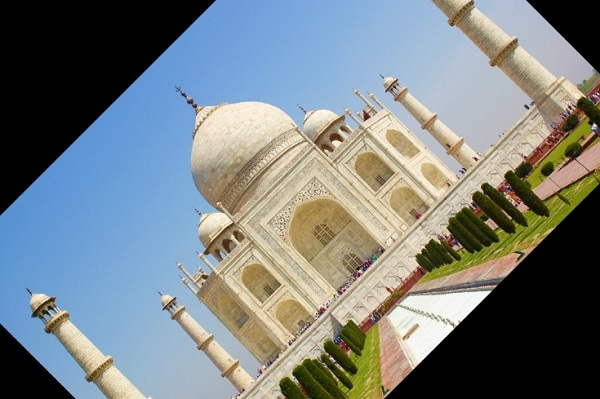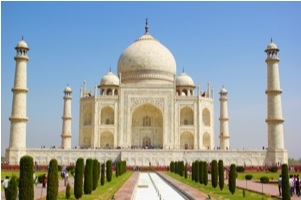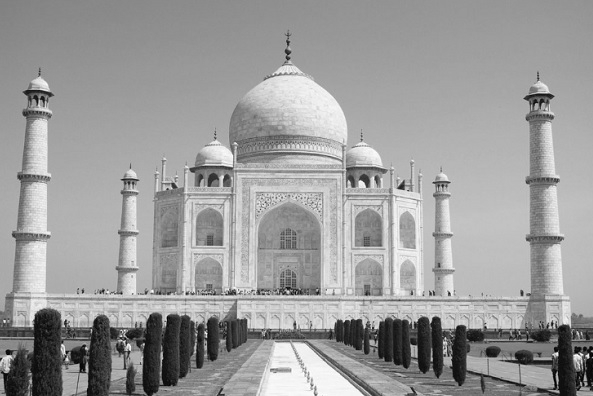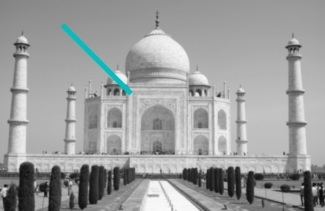# Image processing in Python?

Python provides lots of libraries for image processing, including −

• OpenCV − Image processing library mainly focused on real-time computer vision with application in wide-range of areas like 2D and 3D feature toolkits, facial & gesture recognition, Human-computer interaction, Mobile robotics, Object identification and others.

• Numpy and Scipy libraries − For image manipuation and processing.

• Sckikit − Provides lots of alogrithms for image processing.

• Python Imaging Library (PIL) − To perform basic operations on images like create thumnails, resize, rotation, convert between different file formats etc.

In this section we are going to see some basics of image processing in python.

## Install required library

Our first step will be to install the required library, like openCV, pillow or other which we wants to use for image processing. We can use pip to install the required library, like −

\$pip install pillow

That's it: now we can play with our image.

## Image: Open() and show()

First, open the file/image and show. You can rotate the image while showing like below −

#Import required library
from PIL import Image

#Open Image
im = Image.open("TajMahal.jpg")

#Image rotate & show
im.rotate(45).show()

## OutputAs the above variable im, is a pillow object. We can retreive some information about the opened image −

>>> im
<PIL.JpegImagePlugin.JpegImageFile image mode = RGB size = 1000x667 at 0x65AB990<
>>> im.size
(1000, 667)
>>> im.format
'JPEG'
>>>

## Convert and Save() Image

We can change the format of image from one form to another, like below −

>>> im.save('TajMahal.png')

Now if we see the folder, we have same image in two different formats.

## Resize-thumbnails()

We can change the size of image using thumbnail() method of pillow −

>>> im.thumbnail ((300, 300))
>>> im.show()

The image will change as follows:## Converting to grayscale image − convert()

We can make the grayscale image from our original colored image.

>>> TajMahal_gray = Image.open('TajMahal.jpg').convert('L')
>>> TajMahal_gray.show()

Where "L" stands for 'luminous'.Above example is from the PIL library of python. We can use other library like open-cv, matplotlib & numpy for image processing. Below are some of the example program to demonstrate the use of much powerful library for image processing.

Showing image in grayscale

#Import required library
import cv2
import numpy as np
from matplotlib import pyplot as plt

cv2.imshow('image',im)
cv2.waitKey(0)
cv2.destroyAllWindows()

## OutputAnother way to write above program with a tick/line to mark the image.

import cv2
import numpy as np
from matplotlib import pyplot as plt

plt.show()